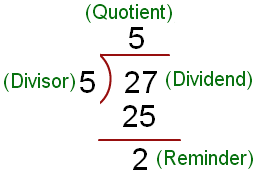# Support Our Site

To ensure we can continue delivering content and maintaining a free platform for all users, we kindly request that you disable your adblocker. Your contribution greatly supports our site's growth and development.

# Python Program To Compute Quotient and Remainder Of Two Numbers

In Division The number which we divide is called the dividend. The number by which we divide is called the divisor. The result obtained is called the quotient. The number left over is called the remainder.## Problem Definition

Create a Python program to compute Quotient and reminder of two given numbers.

## Program

``````divisor = 5
dividend = 27
quotient = dividend//divisor
reminder = dividend % divisor

print("Quotient is", quotient)
print("Reminder is",reminder)``````

#### Output

``````Quotient is 5
Reminder is 2``````

First, the numbers are saved in respective variables then to compute quotient we used the floor division `//` operator that returns the integer value of the quotient and for the reminder, the modulus `%` operator is used. Later we are just printing out the variables.

## Problem Definition

Create a Python program to compute Quotient and reminder of two user-provided numbers in real-time.

## Program

``````divisor = int(input("Enter the divisor"))
dividend = int(input("Enter the dividend"))
quotient = dividend//divisor
reminder = dividend % divisor

print("Quotient is {} and Reminder is {}".format(quotient, reminder))``````

#### Output

``````Enter the divisor 5
Enter the dividend 27
Quotient is 5 and Reminder is 2``````

Here we are taking input from the user using the  Python's built-in `input()` method then we are converting it to an integer using the `int()` method because `input()` returns the objects as a string object.

Later we are performing the arithmetic operation and printing out the results using string formatting.

PROGRAMS

# Latest Articles

Latest from djangocentral

## How to Use Subquery() in Django With Practical Examples

In the realm of web development, Django stands as a powerful and versatile framework for building robust applications. One of the key aspects of developing efficient and optimized web applications is handling database queries effectively. In this article…

## DRF Serializer: Handling OrderedDict and Converting It to a Dictionary or JSON

In Django Rest Framework (DRF) tests, when you access serializer.data, you might encounter an OrderedDict instead of a regular dictionary. This behavior is intentional and reflects the design of DRF's serialization process.Understanding the Problem The u…

## Django Rest Framework CheetSheet: Mastering API Development

Django Rest Framework (DRF) is a powerful toolkit that makes building robust and scalable web APIs with Django a breeze. Whether you're a seasoned Django developer or a newcomer, having a comprehensive cheat sheet at your disposal can be a game-changer. …